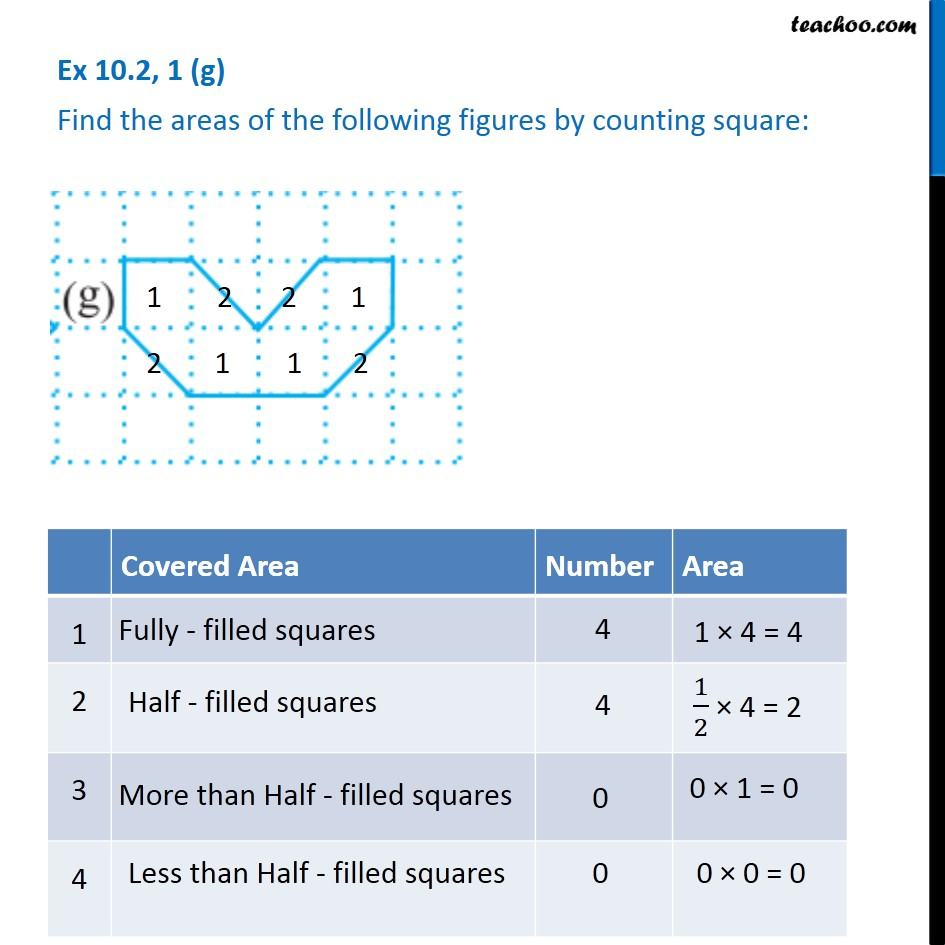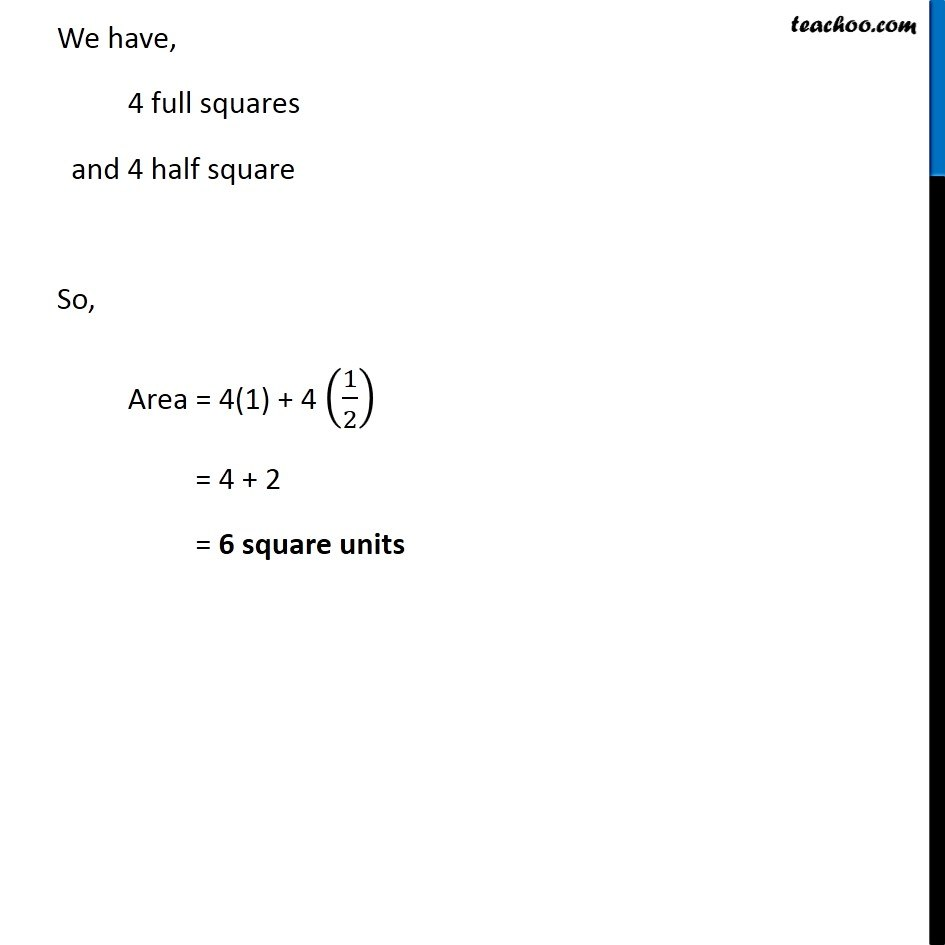1. Chapter 10 Class 6 Mensuration
2. Concept wise
3. Finding area by counting squares

Transcript

Ex 10.2, 1 (g) Find the areas of the following figures by counting square: Covered Area Number Area 1 Fully filled squares 4 1 × 4 = 4 2 Half filled squares 4 1/2 × 4 = 2 3 More than Half filled squares 0 0 × 1 = 0 4 Less than half filled squares 0 0 × 0 = 0 We have, 4 full squares and 4 half square So, Area = 4(1) + 4 (1/2) = 4 + 2 = 6 square units

Finding area by counting squares